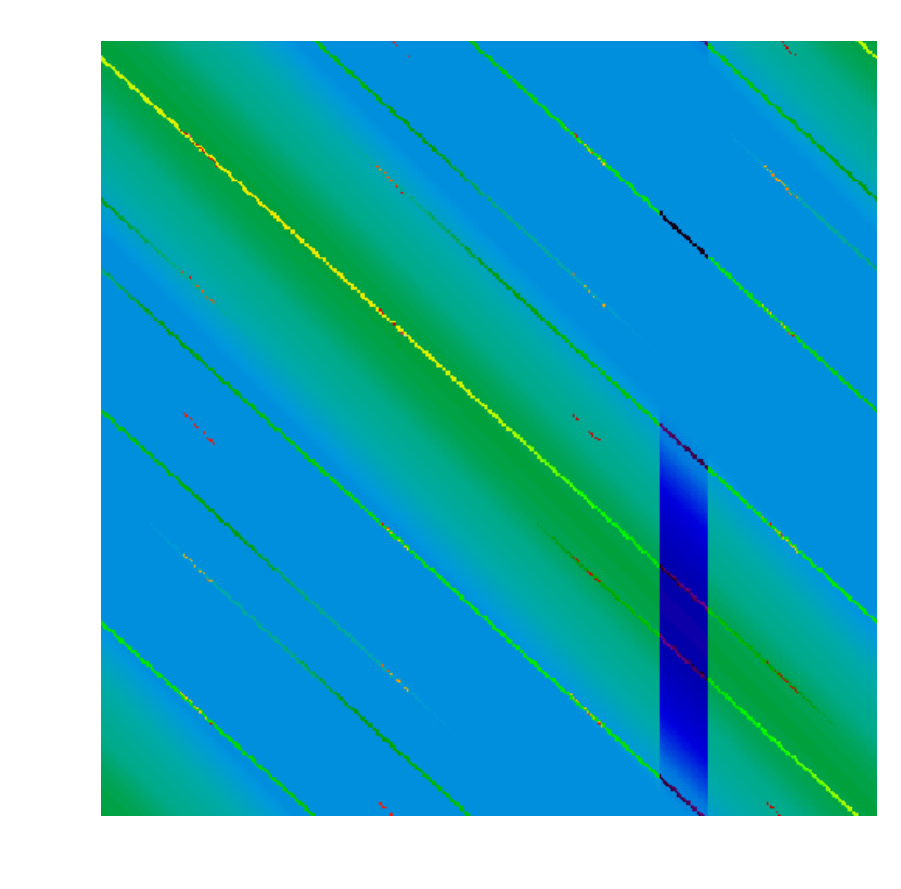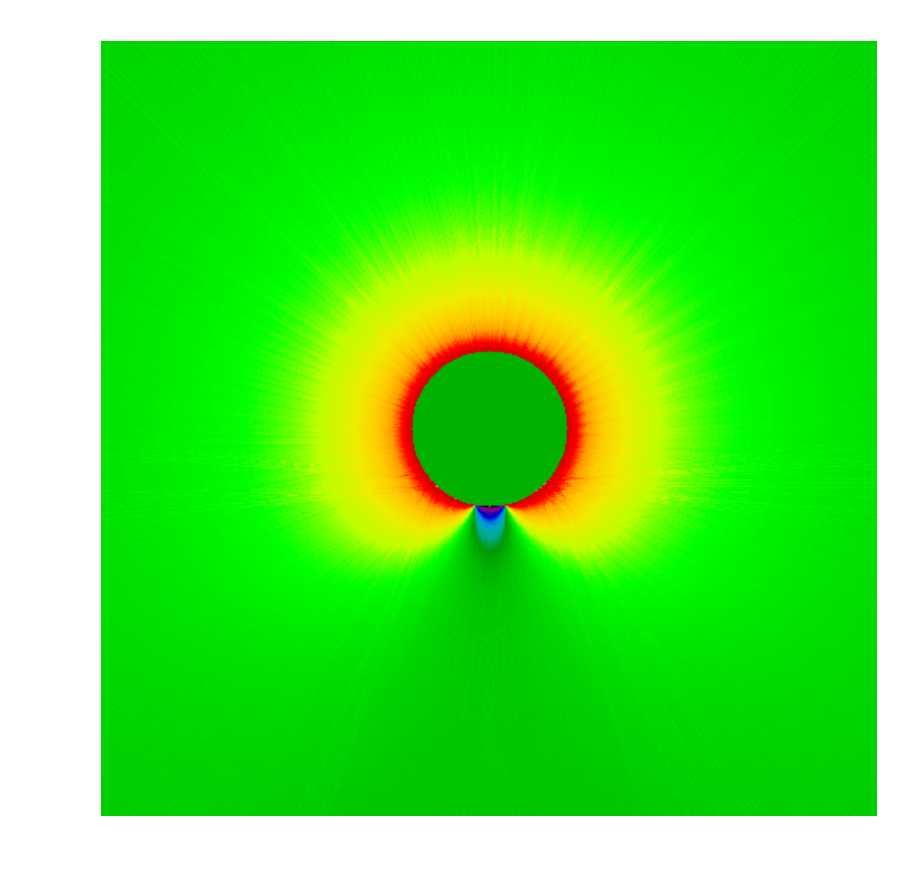# The Porus Mirror Problem, Part 2

## Propagation

From last time, we know the quantity of light that escaped at each location and direction around the unit circle. We want to find how this translates into quantities of light at any point away from the circle. This situation can be modeled as so:The basic idea is to find for any given $$\alpha, r$$ the set of possible $$\phi, \theta$$ values. In any case, it is easiest to eliminate the $$\phi$$ variable via rotation.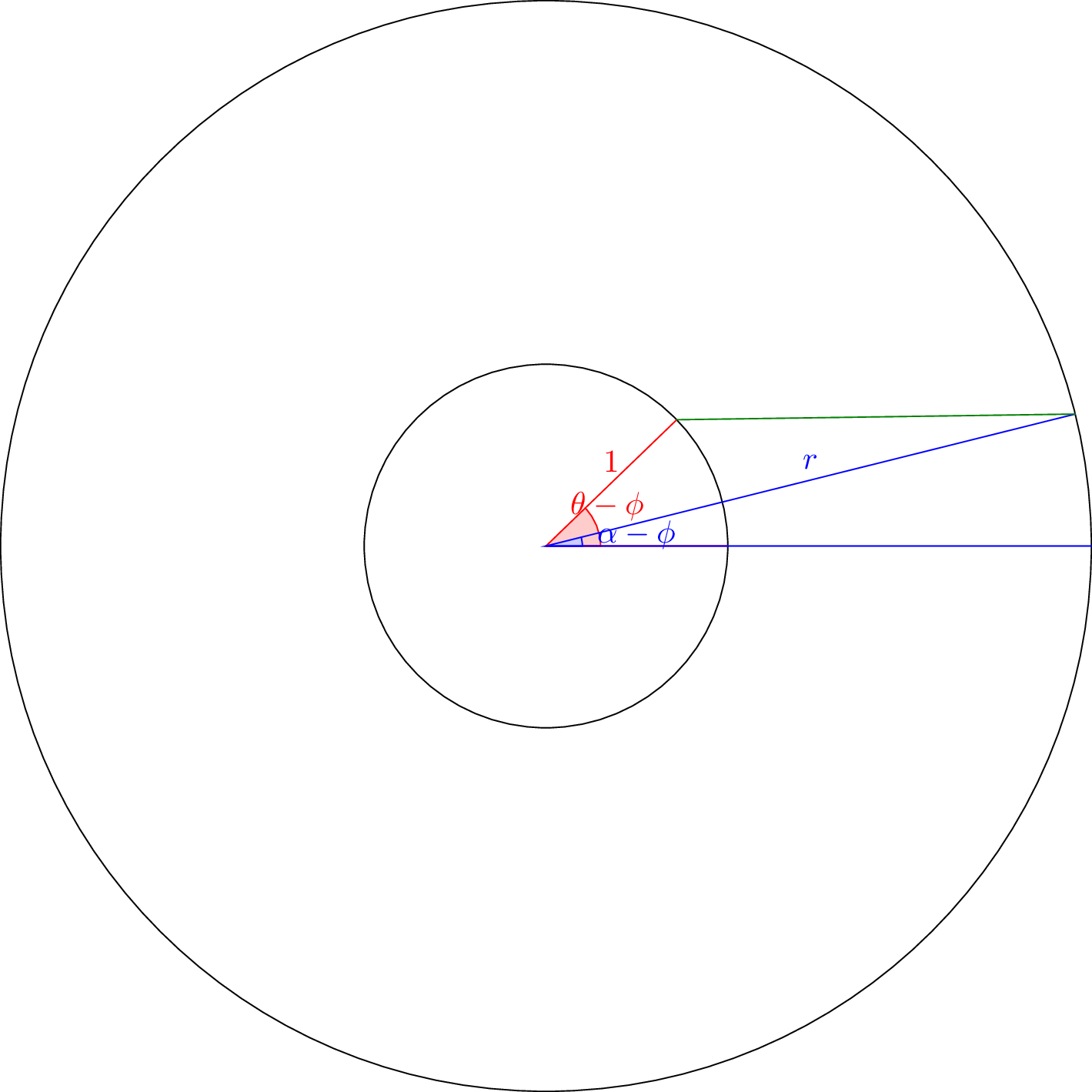Noting that the two angles have the same $$y$$ coordinate, we end up with the equation:

$r\sin(\alpha-\phi) = \sin(\theta-\phi)$

Therefore, we can calculate $$\theta$$ in terms of everything else as

$\theta = \phi + \sin^{-1} \left(r\sin(\alpha-\phi)\right)$

This is defined whenever $$-1 \leq r\sin(\alpha-\phi) \leq 1$$, which is true whenever $$\alpha-\sin^{-1} \frac{1}{r} \leq \phi \leq \alpha + \sin^{-1} \frac{1}{r}$$.

We can therefore calculate the quantity of light at any point as

$E (r, \alpha) = \int_{\alpha-\sin^{-1} \frac{1}{r}}^{\alpha+\sin^{-1} \frac{1}{r}}L\left(\phi, \phi + \sin^{-1} \left(r\sin(\alpha-\phi)\right)\right)\text{d}\phi$

## A constant transparency

As might be expected, a constant transparency yields a graph of $$L$$ perfectly symmetrical across $$\phi = \theta$$: it is only a function of $$\phi - \theta$$. (The following graph has $$\theta$$ on the $$x$$ axis and $$\phi$$ on the $$y$$.)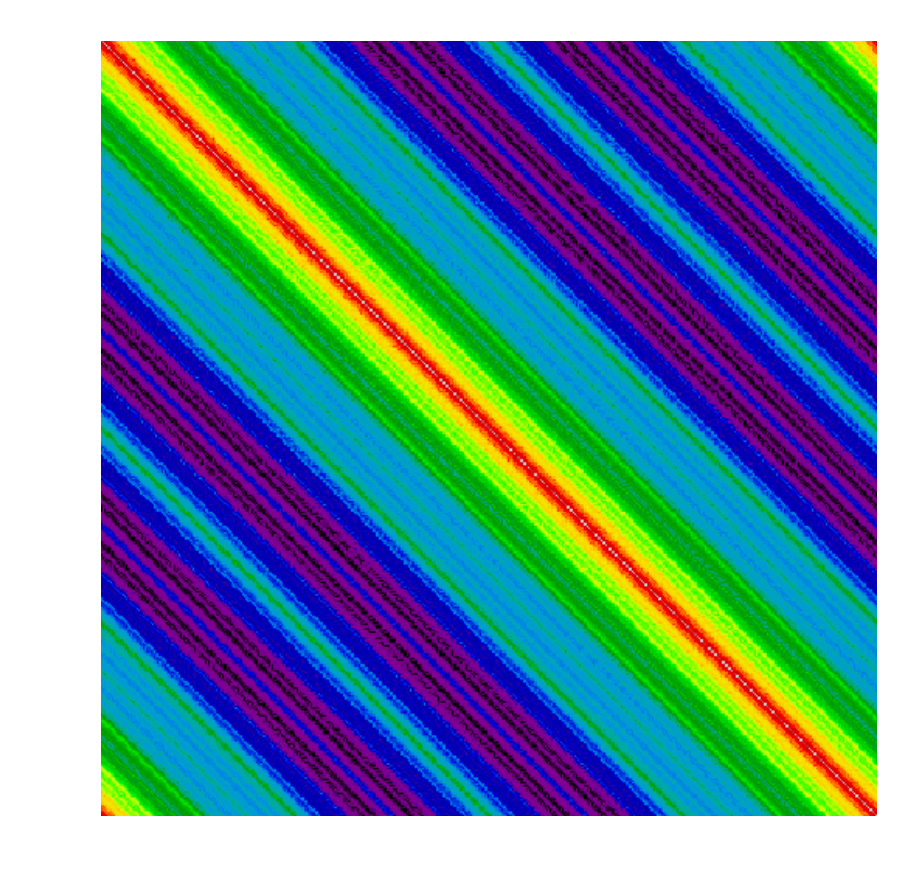This yields the symmetrical circular pattern of energy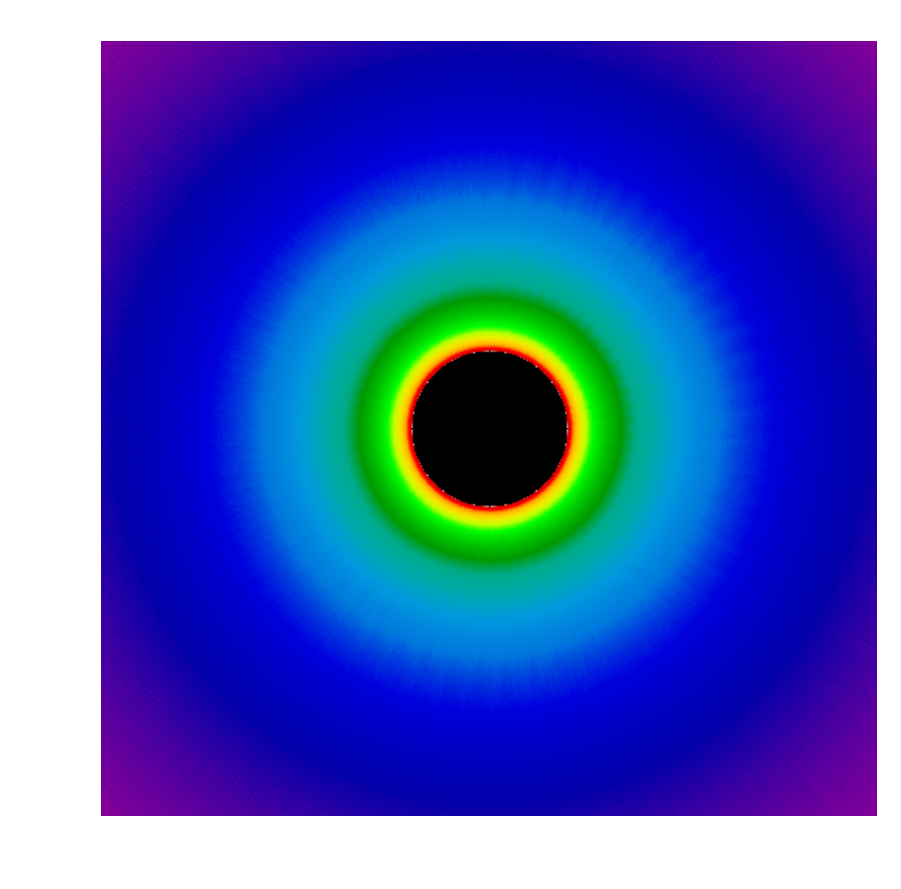## Shifted $$\sin$$

To get a less symmetrical pattern, we can shift the $$\sin$$ function into $$[0,1]$$. This function is effectively $$y$$ so results in the top-biased: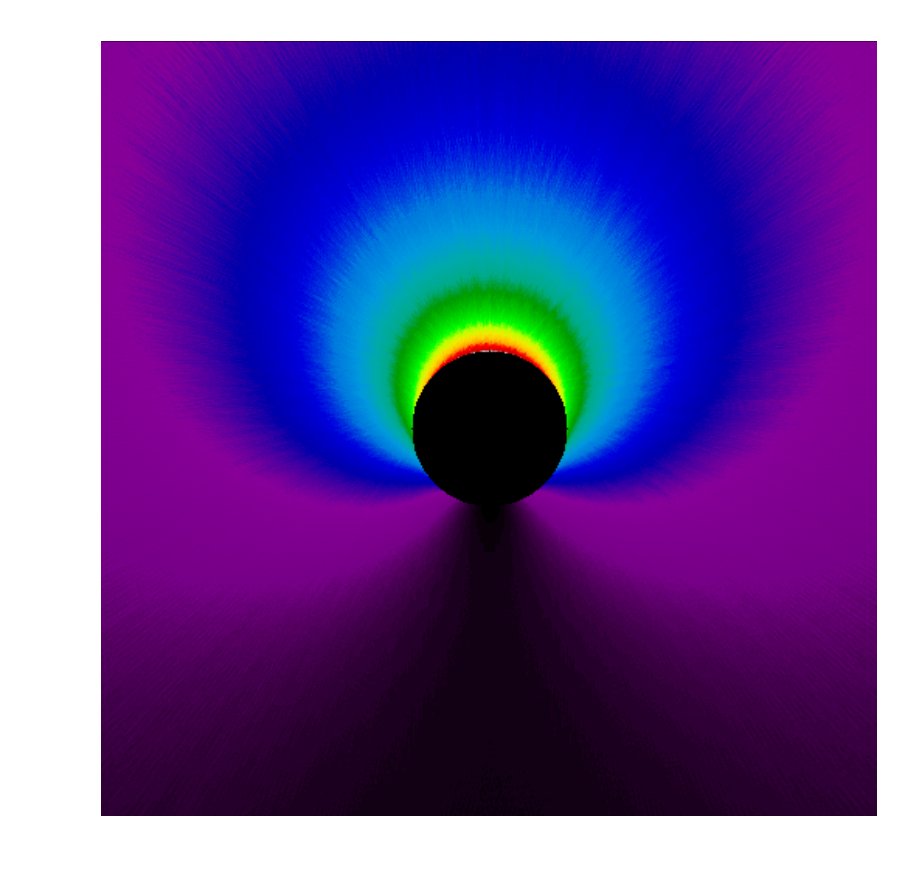## Periodic pattern on surface

Using the 3-period periodic function $$\sin^2 \frac{3\theta}{2}$$, we get a 3-petal flower: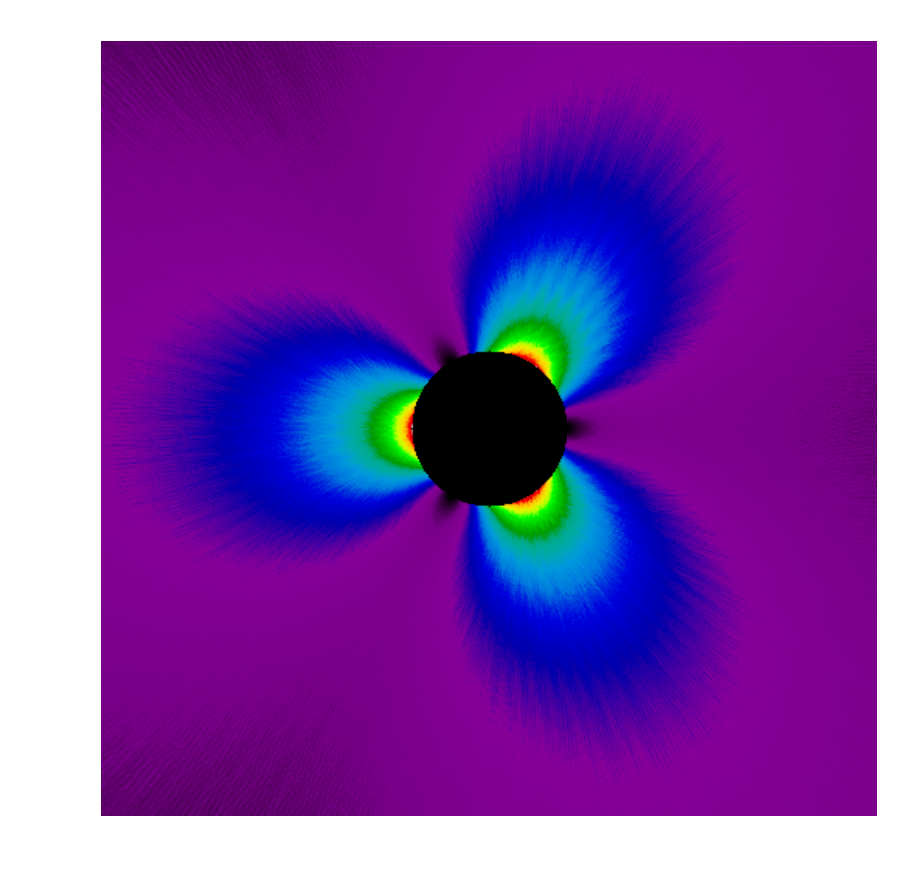## Half-opaque

Making the bottom half of the circle opaque leads to the diagram: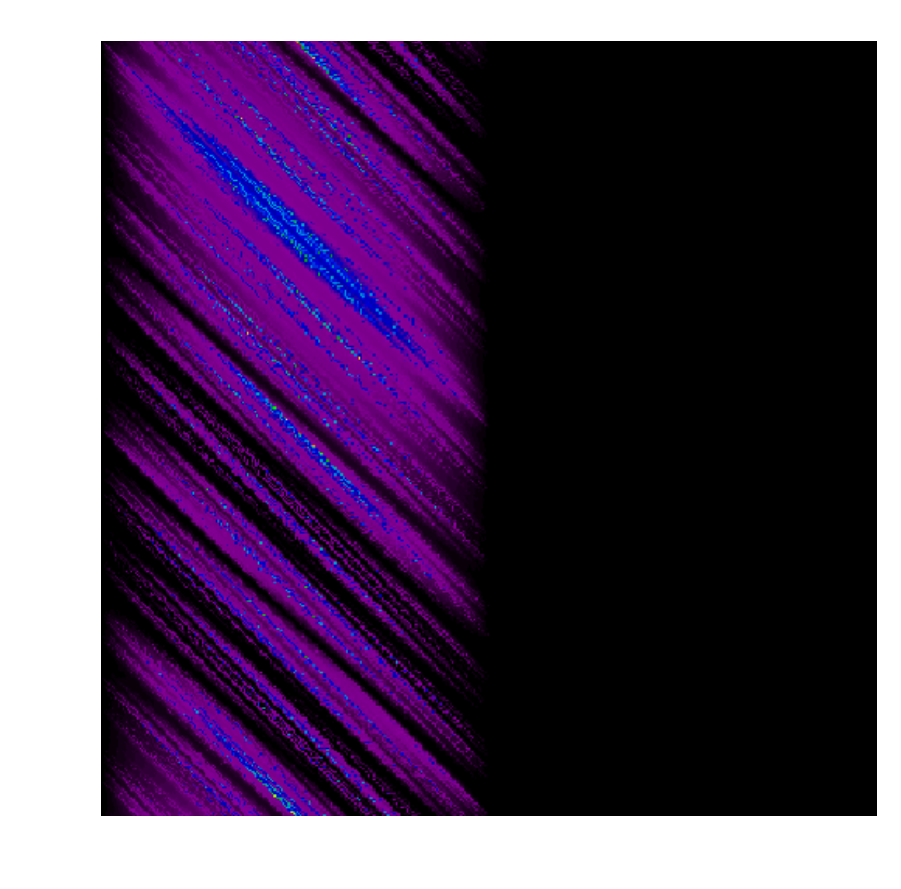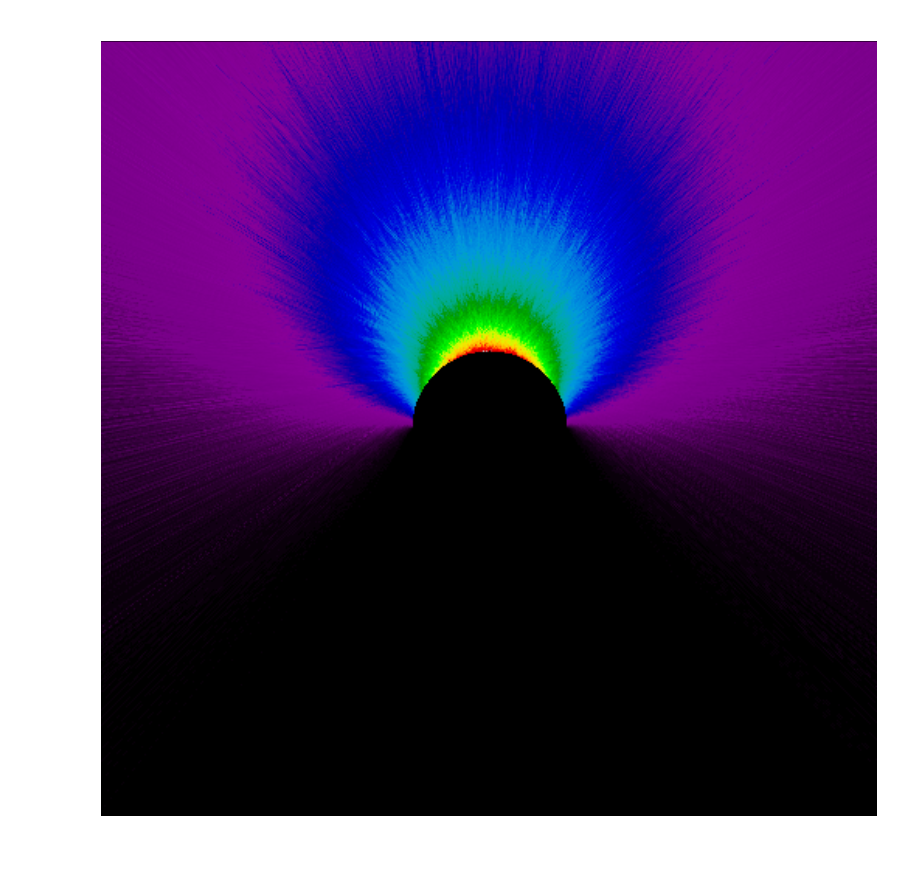## A Tiny Opaque piece

A small opaque piece casts a small shadow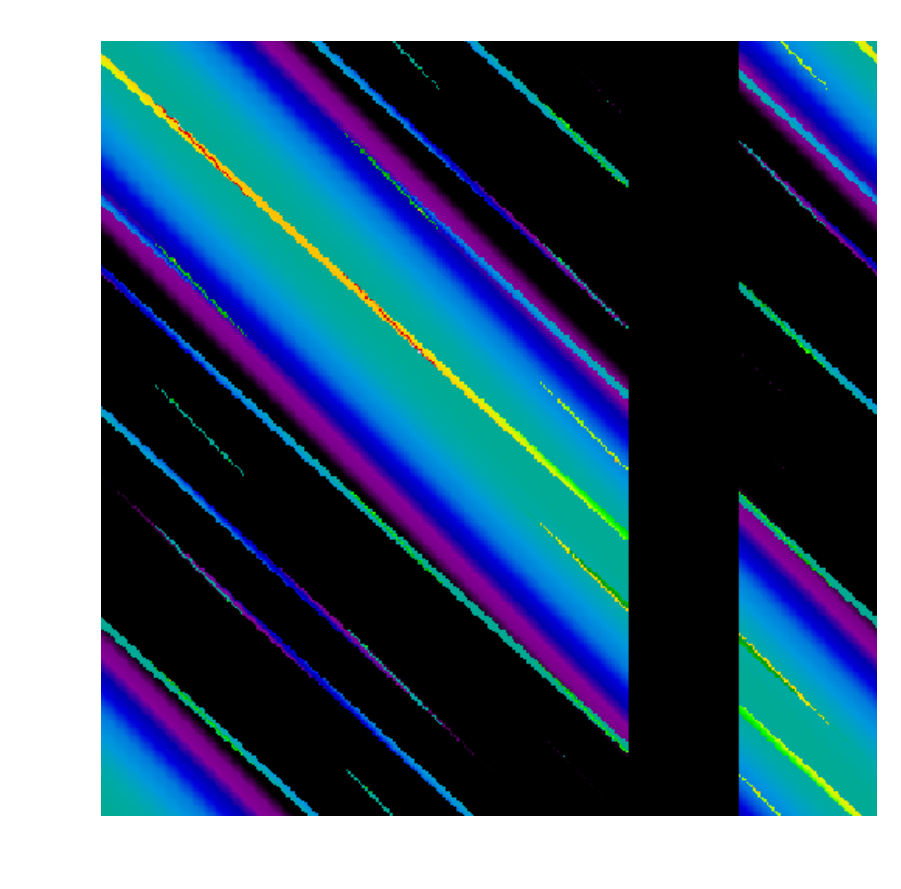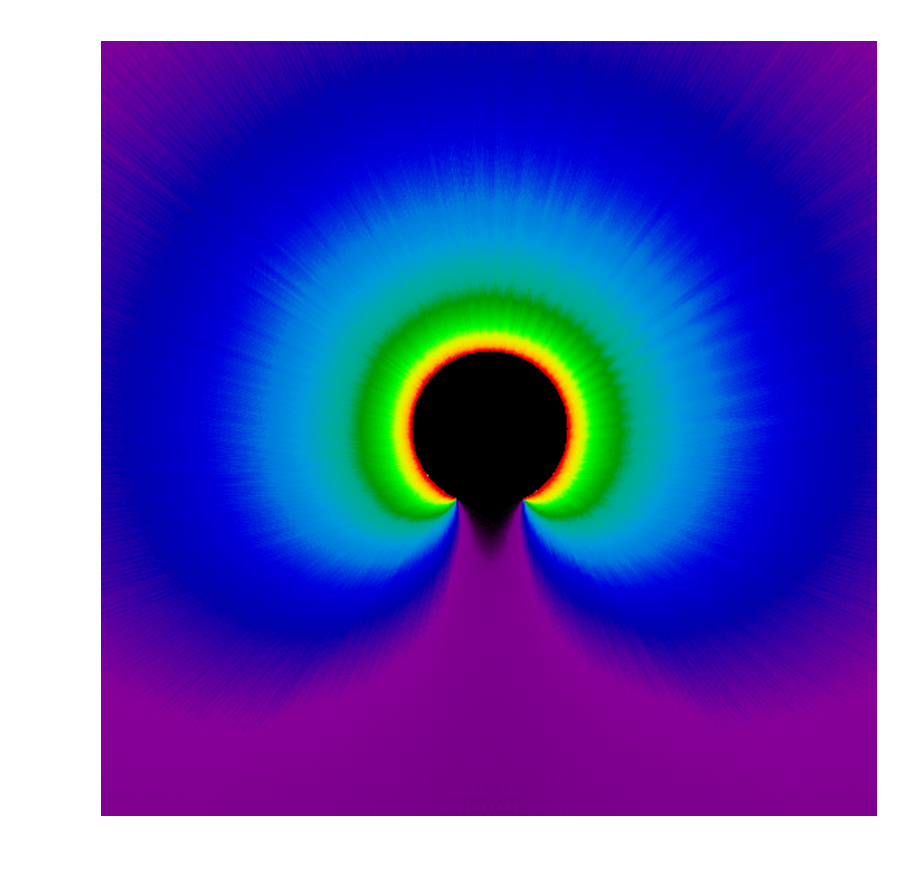## A Single Slit

Because our model is corpuscular, we don’t have diffraction.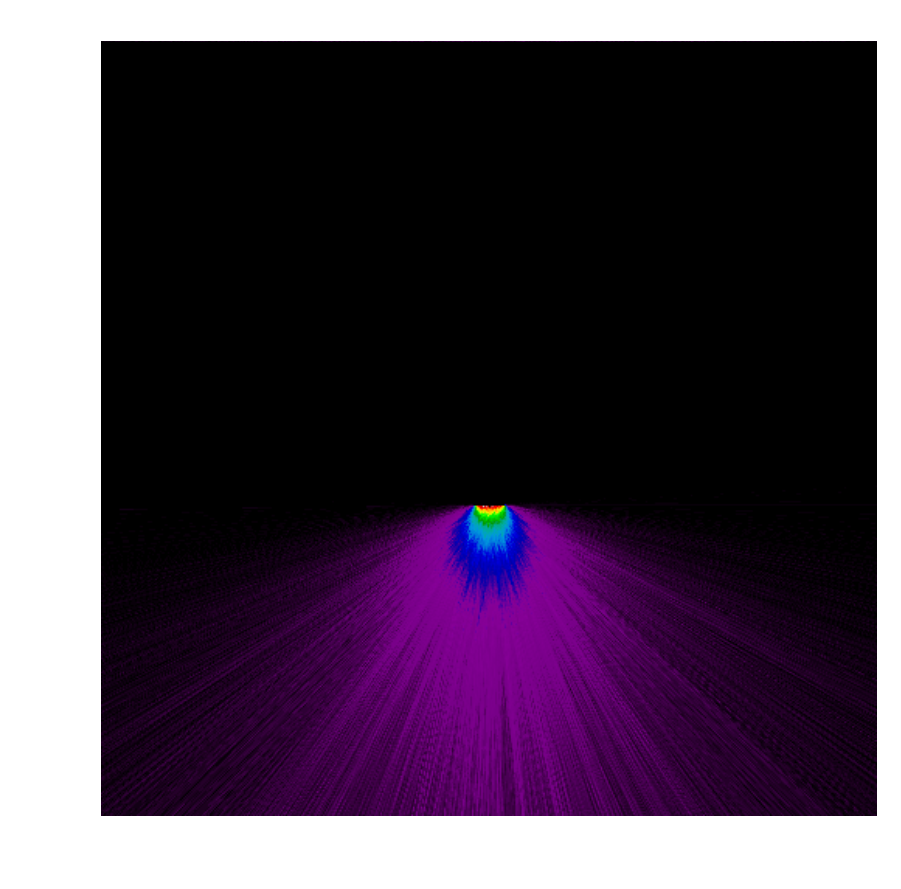## A Double Slit

Again, no diffraction. The pattern that occurs is mostly an aliasing effect.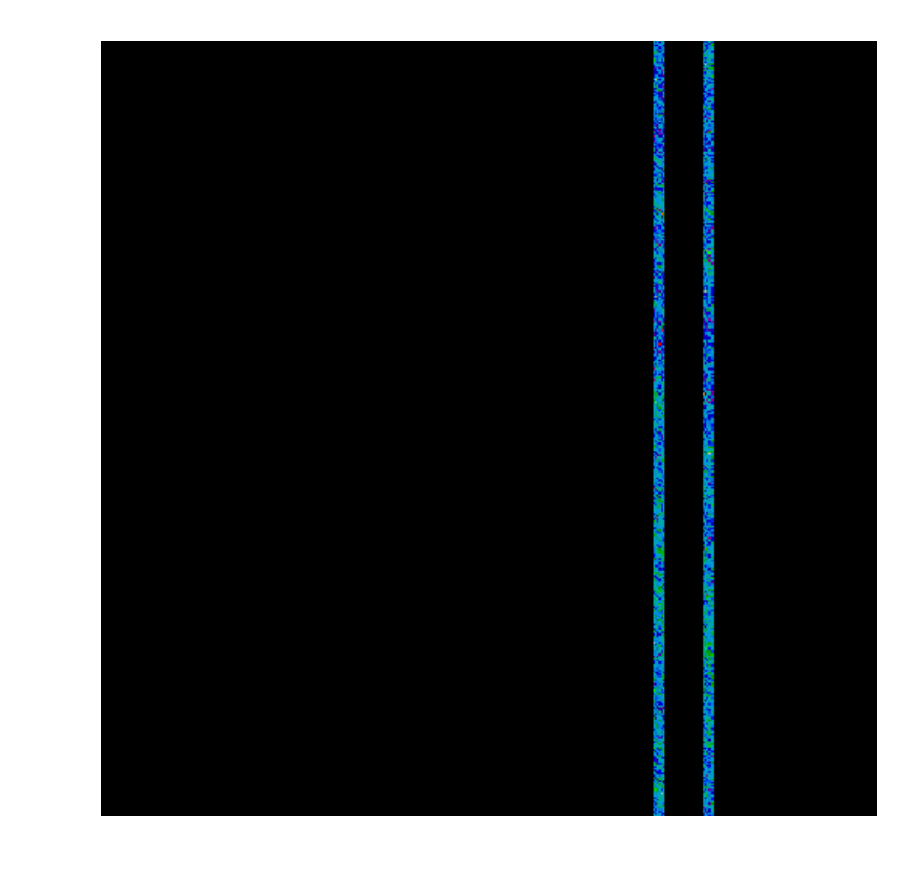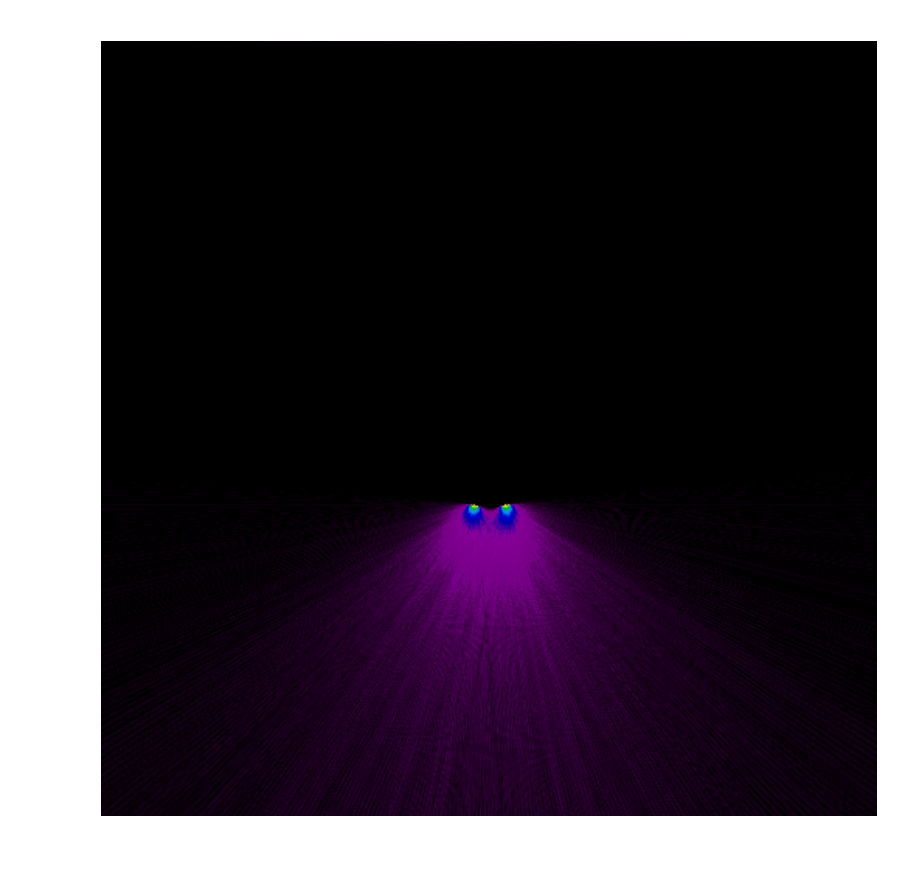## Negative Slit

Our model is flexible enough to allow for “negative transparency”, when “negative light” is projected forwards while extra light is reflected backwards. This is basically just a color inverted version of a small reflector.